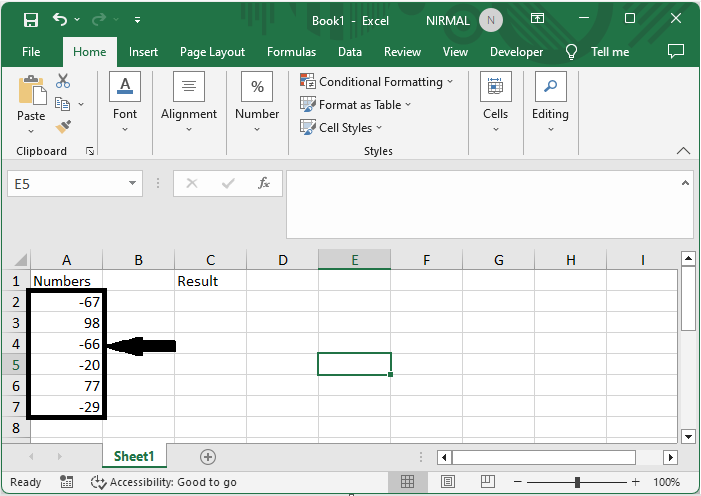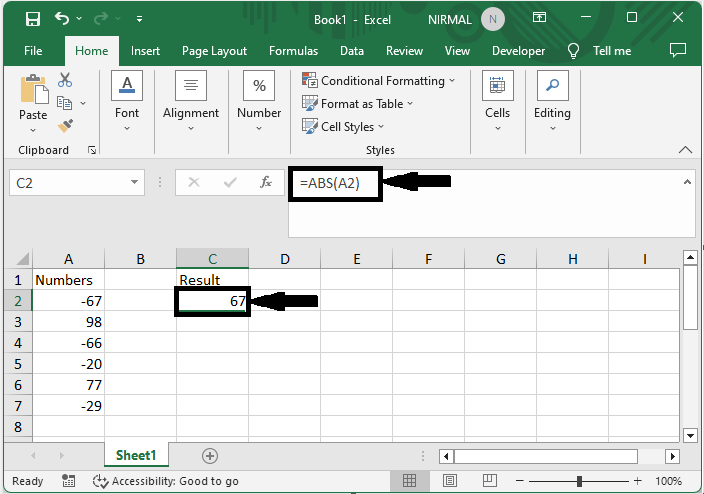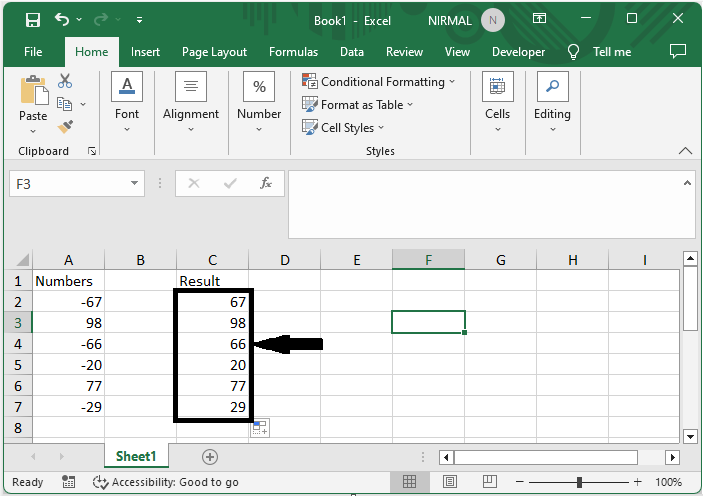# How to Remove Negative Sign From Numbers in Excel?

Excel is a strong programme that is frequently used for financial computations, data analysis, and other tasks. Negative numbers must frequently be converted to positive numbers or have the negative sign removed in order to be used in data manipulation. Using the ABS formula and the auto fill handle, we can finish the task.

We will consider the most straightforward approach to completing the work. In this article, we'll walk you through a variety of ways to complete this work while taking into account various user preferences and scenario scenarios. Regardless of your level of Excel proficiency, we have you covered!

## Remove Negative Sign From Numbers

Here we will first get any one of the values using the formula, then use the autofill handle to complete the task. So let us see a simple process to know how you can remove negative signs from numbers in Excel.

### Step 1

Consider an Excel sheet where you have a range of cells with numbers and some negative numbers, similar to the below image.First, click on an empty cell and enter the formula as =ABS(A2) and click enter to get the first value.

Empty Cell > Formula > Enter.### Step 2

Then drag using the autofill handle to complete the task. Then you will see that the negative sign has been removed.This is how you can remove a negative sign from a number in Excel.

Note

• This method will generate a new set of values in the sheet.

• If you do not want to print data in the cells, you can format the cells to complete the task.

## Conclusion

In this tutorial, we have used a simple example to demonstrate how you can remove the negative sign from numbers in Excel to highlight a particular set of data.

Updated on: 08-Sep-2023

2K+ Views